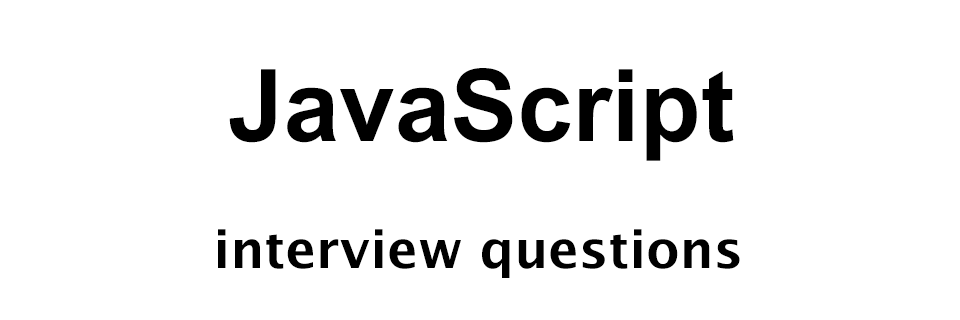# JavaScript Interview Questions - Set 2

JavaScript Interview Questions## Q1: Create the single line and multiline comments in JavaScript

For single line comments we use the `//` followed by the comment.

For multi line comments we enclose the comment lines in `/*` and `*/`.

Example:

``````// This is a single line comment

/**
* This is a multi
* line comment
*/
``````

## Q2: What is the difference between `==` and `===` operators in JavaScript?

The `==` operator checks only the value for equality. Whereas, `===` checks both the value and the data type.

Example:

The following code in JavaScript will give us true.

``````console.log(3 == "3");
``````

The following code in JavaScript will give us false. As 3 and "3" though are same value but are of different types.

``````console.log(3 === "3");
``````

## Q3: How will you select an element in the page having id "awesome" using JavaScript?

To select an element by id we use the `document.getElementById();` method.

In the following code we are selecting the element having id "awesome".

``````var el = document.getElementById("awesome");
``````

## Q4: How will you select elements in a page having class "super" using JavaScript?

For this we will use the `document.getElementsByClassName()` method.

In the following example we are selecting all the elements in the page having class "super".

``````var elems = document.getElementsByClassName('super');
``````

## Q5: Write JavaScript code to get the text of a button having id "my-button"

For this we will first select the button by id. Then we will get the text using the `innerText` property.

``````var el = document.getElementById('my-button');
var text = el.innerText;
console.log(text);
``````

## Q6: How will you set the text of a button using JavaScript?

For this we can use the `innerText` property and set it to the required value.

In the following example we are selecting a button having id 'super-btn' and setting the text to 'Super button'.

``````var el = document.getElementById('super-btn');
el.innerText = 'Super button';
``````

## Q7: Dynamically create a paragraph having some text inside a div having id 'sample-div' using JavaScript

For this we will first get the div by id 'sample-div'. Then we will create a paragraph and insert it inside the div using the `innerHTML` property.

``````// get the div by id
var div = document.getElementById('sample-div');

// create a paragraph
var p = "<p>This is a sample paragraph.</p>";

// insert the paragraph in the div
div.innerHTML = p;
``````

## Q8: Create a greetings function in JavaScript that will print your name when it is called

We can create a function by the name `printName()` having parameter `name`.

``````function printName(name) {
console.log('Hello ' + name + '!');
}

printName('Yusuf Shakeel');
``````

## Q9: How will you check if a given value is not a number in JavaScript?

For this we can use the `isNaN()` method which returns `true` if the value passed is not a number otherwise, false.

The following code will print true as the value stored in variable x is not a number.

``````var x = 'hello';
console.log(isNaN(x));
``````

## Q10: Write the output of the following JavaScript code

``````function foo(x) {
var result = x * 10 + "20";
console.log(result);
}

foo(2);
``````

The above code will print `2020`.

Explanation:

We are passing 2 to the `foo` function which is assigned to the variable x. Then we are multiplying 10 to x. So, we get 20.

Next, we are adding the number 20 to the string "20".

The + operator concatenates the number 20 and the string "20" and the result is a string. So, the final result is 2020.